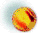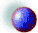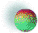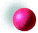Mathematics and Statistics Resources
ProductsStatistical Process ControlMathematics for Visualization (Mapple V)Wolfram's Research MathematicaThe Mathematica BookstoreStatistical SolutionsMATLAB

• http://www.mathtools.com  - Complementary products for MATLAB, like MIDEVA (fast MATLAB replacement), MATCOM (Compiler for MATLAB), Visual MATCOM (integrate m-files into Visual C++) and others, all available for download.StatisticsJournal of Satistical EducationThe United States Census BureauHistory of Mathematics Links

NOTE: The user is encouraged to verify the accuracy and reliability of any material drawn from the listed sources.
• The Mathematical Museum - History Wing at ZIB (DE)
•      Contains a LARGE COLLECTION of links to other mathematical Web resources!
• MacTutor History of Mathematics Archive at St Andrew's University (UK)
•      A wide range of material, includes over 1000 biographies of mathematicians -- NB: the information available here is not always reliable!
• Biographies of Women Mathematicians at Agnes Scott College (USA)
•      Some detailed biographies of women mathematicians as well as many biographical sketches, this collection is growing.
• History of Mathematics at Clark University (USA)
•      Extensive chronology of mathematicians, includes non-western mathematics.
• The History of Mathematics at Trinity College (Ireland)
•      Contains excerpts from W. W. Rouse Ball's A short account of the history of mathematics
• The Mathematical Quotations Server at Furman University (USA)
•      Quotations by mathematicians and on mathematics
• Favorite Mathematical Constants
•      Information on well-known as well as some more obscure constants.
• The Hooke Museum of the History of Science at Oxford (UK)
• Other Math ResourcesMortgage Calculator -- among other things, this site will calculate a full amortization table!

Math Archives -- a resource for teachers as well as mathematicians

Tutorials/White PapersAn Introduction to Mathematisc for Business and Everyday Life